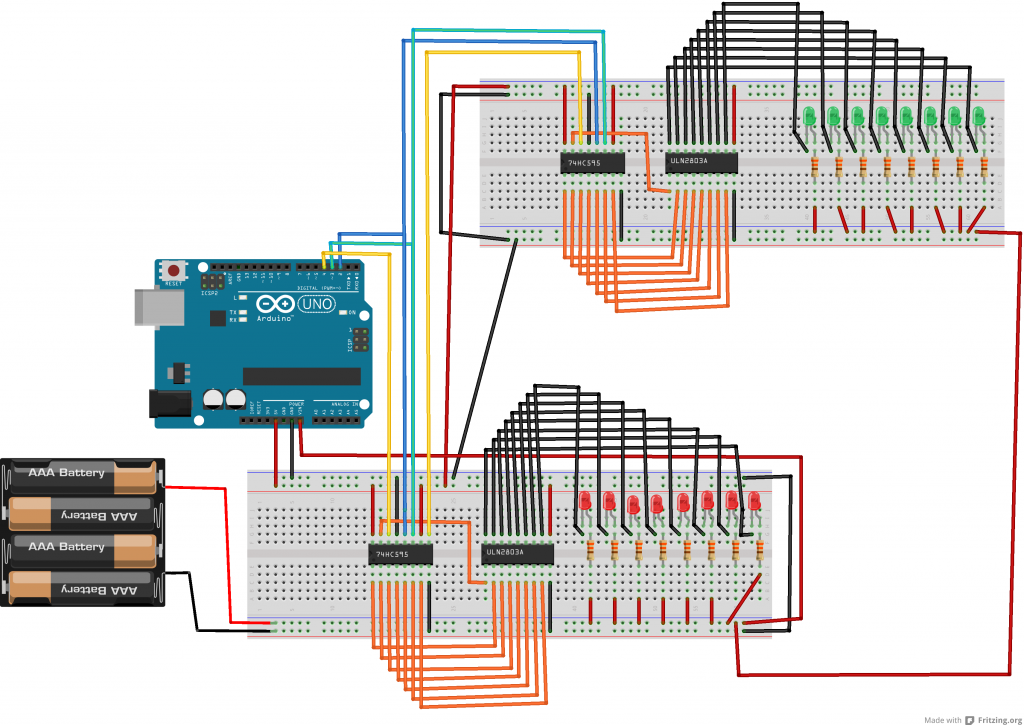A video illustrating the previous article .
The red is a bit flashy, sorry for that…

In a previous article, we had used a 74HC595 to control a ULN2803.
This enabled us to deal with 8 LED’s.

Lets now cascade two 74HC595 to deal with 16 LED’s.
To do this, we will use the serial output of 74HC595 #1 to the serial input of 74HC595 #2.

Here below the schema.Here below the arduino sketch.
Note that we use two arrays, and that we go up and down in each array.

```//the pins we are using
int latchPin = 2;
int clockPin = 3;
int dataPin = 4;

byte dataArrayA;
byte dataArrayB;

void setup() {
//set all the pins used to talk to the chip
//as output pins so we can write to them
pinMode(latchPin, OUTPUT);
pinMode(clockPin, OUTPUT);
pinMode(dataPin, OUTPUT);

dataArrayA = 0xFF; //11111111
dataArrayA = 0xFE; //11111110
dataArrayA = 0xFC; //11111100
dataArrayA = 0xF8; //11111000
dataArrayA = 0xF0; //11110000
dataArrayA = 0xE0; //11100000
dataArrayA = 0xC0; //11000000
dataArrayA = 0x80; //10000000
dataArrayA = 0x00; //00000000

dataArrayB = 0xFF; //11111111
dataArrayB = 0xFE; //11111110
dataArrayB = 0xFC; //11111100
dataArrayB = 0xF8; //11111000
dataArrayB = 0xF0; //11110000
dataArrayB = 0xE0; //11100000
dataArrayB = 0xC0; //11000000
dataArrayB = 0x80; //10000000
dataArrayB = 0x00; //00000000

}

void loop() {
for (int i = 0; i < 9; i++) {
digitalWrite(latchPin, LOW);
shiftOut(dataPin, clockPin, MSBFIRST, dataArrayA[i]);
shiftOut(dataPin, clockPin, MSBFIRST, dataArrayB[i]);
digitalWrite(latchPin, HIGH);
delay(100);
}

for (int i = 8; i >= 0; i--) {
digitalWrite(latchPin, LOW);
shiftOut(dataPin, clockPin, MSBFIRST, dataArrayA[i]);
shiftOut(dataPin, clockPin, MSBFIRST, dataArrayB[i]);
digitalWrite(latchPin, HIGH);
delay(100);
}

}
```

I discussed WinFE some weeks ago here.

It got updated with the following significant features (amongst others) : SysWOW64 and UEFI support. See here.